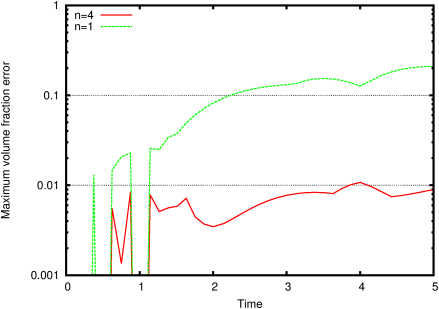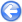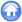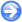### 3.3PASS: Rotation of a straight interface

Author
Stéphane Popinet
Command
gerris2D rotate.gfs
Version
110920
Required files
rotate.gfv cells.gfv error.ref n1-0.gnu n1-1.gnu n1-2.gnu n1-5.gnu error.n1
Running time

A straight interface is advected by a pure shear flow. The exact solution is simply a rotation of the interface around the center of the box. Note that both the interface and the velocity field should be described exactly by a second-order method.

Figure 41 illustrates what happens when using a VOF scheme to follow the interface. The green segments are the VOF-reconstructed interface obtained when using a "naive" Eulerian PLIC method. The red segments are the VOF-reconstructed interface obtained when using a "multiband" Eulerian PLIC method (with n = 4 bands).

Figure 42 illustrates the evolution of the error with time. For t=1 the interface is at 45 degrees and the errors in fluxes cancel out exactly.

 Figure 41: Evolution of the VOF interface (green n = 1, red n = 4) in a shear flow. (a) t=0, (b) t=1, (c) t=2, (d) t=5.
 (a)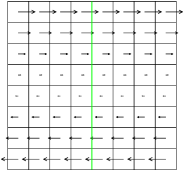(b)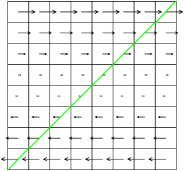(c)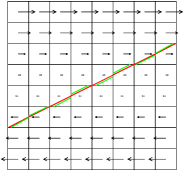(d)Figure 42: Volume fraction error as a function of time.